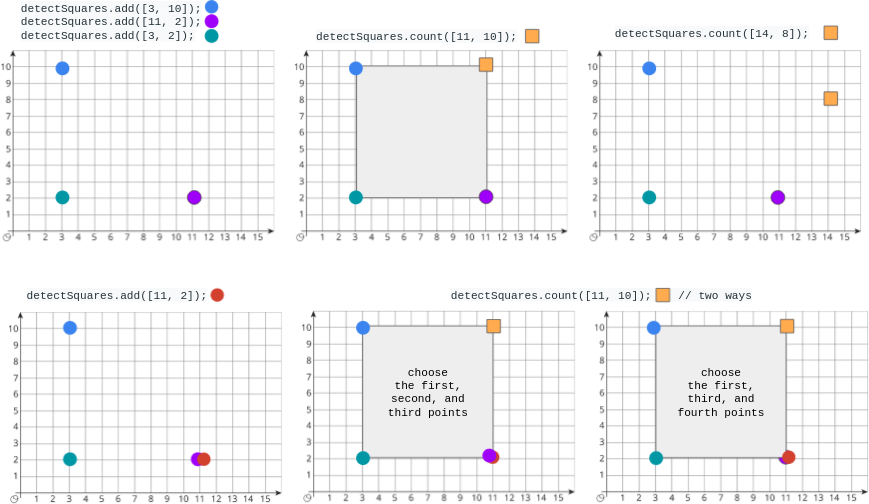# Detect Squares LeetCode Solution

## Problem Statement

Detect Squares LeetCode Solution – You are given a stream of points on the X-Y plane. Design an algorithm that:

• Adds new points from the stream into a data structure. Duplicate points are allowed and should be treated as different points.
• Given a query point, counts the number of ways to choose three points from the data structure such that the three points and the query point form an axis-aligned square with a positive area.

An axis-aligned square is a square whose edges are all the same length and are either parallel or perpendicular to the x-axis and y-axis.

Implement the `DetectSquares` class:

• `DetectSquares()` Initializes the object with an empty data structure.
• `void add(int[] point)` Adds a new point `point = [x, y]` to the data structure.
• `int count(int[] point)` Counts the number of ways to form axis-aligned squares with points `point = [x, y]` as described above.

Example 1:Input

```["DetectSquares", "add", "add", "add", "count", "count", "add", "count"]
[[], [[3, 10]], [[11, 2]], [[3, 2]], [[11, 10]], [[14, 8]], [[11, 2]], [[11, 10]]]
```

Output

```[null, null, null, null, 1, 0, null, 2]

```

Explanation

```DetectSquares detectSquares = new DetectSquares();
detectSquares.count([11, 10]); // return 1. You can choose:
//   - The first, second, and third points
detectSquares.count([14, 8]);  // return 0. The query point cannot form a square with any points in the data structure.
detectSquares.count([11, 10]); // return 2. You can choose:
//   - The first, second, and third points
//   - The first, third, and fourth points
```

Constraints:

• `point.length == 2`
• `0 <= x, y <= 1000`
• At most `3000` calls in total will be made to `add` and `count`.

## Approach

### Idea:

Given p1, try all points p3 (p1 and p3 form diagonal)

• To compute `count(p1)`:
• First, We try all points `p3` which together with `p1,` form the diagonal of the non-empty square. This means `abs(p1.x-p3.x) == abs(p1.y-p3.y) && abs(p1.x-p3.x) > 0`
• Secondly, Since we have 2 points `p1` and `p3`, we can form a square by computing the positions of the 2 remaining points `p2``p4`.
• Thirdly, because of the above`p2 = (p1.x, p3.y)`
• Lastly, because of the above`p4 = (p3.x, p1.y)`## Code

### C++ Program of Detect Squares

```class DetectSquares {
public:
int cntPoints = {};
vector<pair<int, int>> points;

cntPoints[p][p]++;
points.emplace_back(p, p);
}

int count(vector<int> p1) {
int x1 = p1, y1 = p1, ans = 0;
for (auto& [x3, y3] : points) {
if (abs(x1-x3) == 0 || abs(x1-x3) != abs(y1-y3))
continue;
ans += cntPoints[x1][y3] * cntPoints[x3][y1];
}
return ans;
}
};```

### Java Program of Detect Squares

```class DetectSquares {
int[][] cntPoints = new int;
List<int[]> points = new ArrayList<>();

cntPoints[p][p] += 1;
}

public int count(int[] p1) {
int x1 = p1, y1 = p1, ans = 0;
for (int[] p3 : points) {
int x3 = p3, y3 = p3;
if (Math.abs(x1-x3) == 0 || Math.abs(x1-x3) != Math.abs(y1-y3))
continue;
ans += cntPoints[x1][y3] * cntPoints[x3][y1];
}
return ans;
}
}```

### Python Program of Detect Squares

```class DetectSquares:
def __init__(self):
self.cntPoints = Counter()

def add(self, point: List[int]) -> None:
self.cntPoints[tuple(point)] += 1

def count(self, point: List[int]) -> int:
ans = 0
x1, y1 = point
for (x3, y3), cnt in self.cntPoints.items():
if abs(x1 - x3) == 0 or abs(x1 - x3) != abs(y1 - y3):
continue
ans += cnt * self.cntPoints[(x1, y3)] * self.cntPoints[(x3, y1)]
return ans```

## Complexity Analysis for Detect Squares LeetCode Solution

### Time Complexity

To begin with, the time complexity of the above code is O(1) because no matter how large our input is, we are still going to be running the same functions the same amount of times

### Space Complexity

To end, the space complexity of the above code is O(1) because since we are doing only the same functions a constant amount of times, the space will also always be constant

Translate »